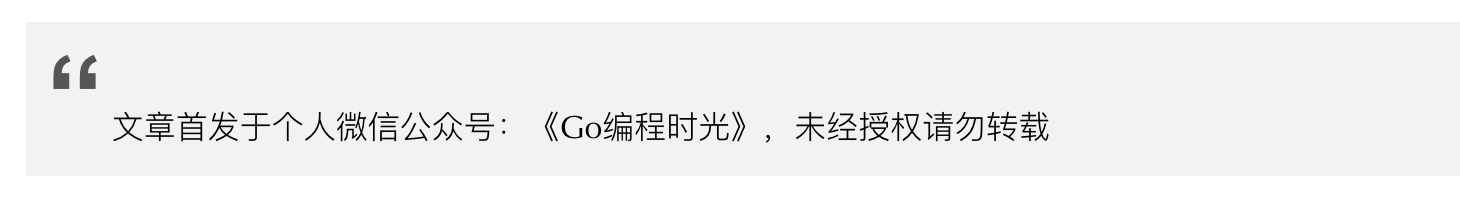# 6.2 单元测试：如何进行单元测试？¶## 1. 单元测试¶

math.go

```package math

return x+y
}
```

math_test.go

```package math

import "testing"

}
```

```\$ go test .
ok      _/home/wangbm/golang/math   0.003s
```

1. 单元测试代码的 go文件必须以`_test.go`结尾，而前面最好是被测试的文件名（不过并不是强制的），比如要测试 math.go 测试文件名就为 math_test.go

2. 单元测试的函数名必须以`Test`开头，后面直接跟要测试的函数名，比如要测试 Add函数，单元测试的函数名就得是 TestAdd

3. 单元测试的函数必须接收一个指向`testing.T`类型的指针，并且不能返回任何值。

## 2. 表组测试¶

```package math

import "testing"

if sum == 3 {
t.Log("the result is ok")
} else {
t.Fatal("the result is wrong")
}

if sum == 6 {
t.Log("the result is ok")
} else {
t.Fatal("the result is wrong")
}
}
```

```\$ go test . -v
TestAdd: math_test.go:8: the result is ok
TestAdd: math_test.go:15: the result is ok
PASS
ok      _/home/wangbm/golang/math   0.003s
```

```package math

import "testing"

type TestTable struct {
xarg int
yarg int
}

tables := []TestTable{
{1,2},
{2,4},
{4,8},
{5,10},
{6,12},
}

for _, table := range tables{
if result == (table.xarg + table.yarg){
t.Log("the result is ok")
} else {
t.Fatal("the result is wrong")
}
}
}
```

```\$ go test . -v